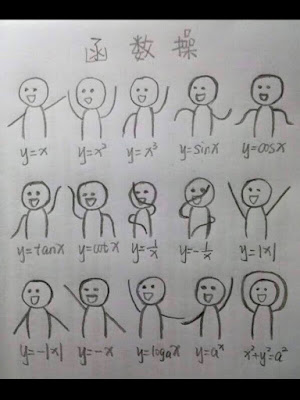## Sunday, November 10, 2019

### Machine Learning Workflow

• Data cleaning
• Missing data
• Outlier
• Others: duplicates, typos, special characters
• Feature engineering
• Strategy for missing data: imputation, mean, median, np.nan, unknown
• Outlier: visualize, demo of linear regression change with outlier, IQR
• Curse of dimensionality: count of columns aka features vs count of rows,
• Data transformation:
• Encoding
• Categorical, one hot encoding, machine readable, ordinal versus independent
• Scaling
• Skewed data
• Sampling
• Stratification
• Class imbalance
• Feature engineering
• Rank transformation

Key concepts
• One hot encoding: a categorical column of three potential values: married, single, divorced will become three separate columns of 1, 0

Core Data structures
• Pytorch tensors
• Tensorflow tensors
• Numpy ndarray
• Pandas dataframe and series

### Data analysis

Data visualization of common math formula.